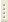06.02.2023Spectroscopy of Semiconductors and Dielectrics

Prof. V.B.Timofeev

Electrons in perfect crystal and energy band concept. Adiabatic approximation. One-electron approximation, Hartree-Fock method. Heitler-London-Heisenberg and Gundt-Bloch methods. Effective mass, positive-hole concept. Energy band structure in germanium, silicon, gallium arsenide. Interband optical transitions and their optical properties. Quantum theory of interband transitions. Relation to optical constants. Theoretical analysis and experimental examples: germanium, layer (sandwich) semiconductor structures. Indirect electron-phonon optical transitions. Two-photon absorption. Drude-Lorentz optical properties of metals. Excitons in crystals. Strong coupling approximation and Fresnel excitons. Wannier-Mott excitons. Effect of external static fields on exciton spectra. Effects of delay and spatial dispersion in exciton resonance region. Collective interaction effects in exciton and nonequilibrium high-density carrier system. Impurity states in dielectrics and semiconductors. Carrier spin and exciton optical orientation in semiconductors. Spin-lattice and spin-spin relaxation. Excitons in magneto-mixed semiconductors. Two-dimensional semiconductor systems. Size quantization spectra in low-dimensional systems. Quantum Hall effect.

Curriculum

1. Electrons in perfect crystal and energy band concept. General formulation of the problem. Adiabatic approximation. One-electron approximation, Hartree-Fock method. Heitler-London-Heisenberg and Gundt-Bloch methods in electron crystal theory.
2. General properties of electron in periodic crystal field. Kronig-Penney model. Effective mass, positive-hole concept. Lattinger Hamiltonian, light and heavy holes. Strong coupling approximation.
3. Energy band structure for specific semiconductors: germanium, silicon, gallium arsenide.
4. Interband optical transitions and their optical properties. General theoretical analysis of interband optical transitions. Main approximations. Electron-phonon interaction Hamiltonian. Quantum theory of interband transitions. Relation to optical constants illustrated by Lorentz oscillator. Dielectric permeability, absorption factors, reflection extinctions, refractive index.
5. Analytical behavior of optical constants in energy spectrum critical points (Van Hove singularity, maximum and minimum points, saddle points). Cases of 3rd, 2nd and 1st measurements. Theoretical analysis and experimental examples: germanium, layer (sandwich) semiconductor structures.
6. Interaction of electrons with deformation and polarized lattice vibrations. Electron polaron. Frelich model of polarized electron-phonon interaction. Indirect electron-phonon optical transitions. Examples: germanium, silicon, gallium phosphide.
7. Multiphoton optical transitions and optical constant structure. Two-photon absorption.
8. Effect of external static actions on semiconductor exciton spectra. Hydrostatic pressure and effect of uniaxial deformation. Effect of static electrical field and Franz-Keldysh effect.
9. Effect of external static magnetic field. Landau oscillator and diamagnetic quantization of electron spectrum. Magnetooptical oscillations in semiconductor absorption spectra. Electron in harmonic potential field (Fock-Darvin model).
10. Drude-Lorentz optical properties of metals. Plasma vibrations and plasma edge structure. Plasma vibration damping (Landau damping). Optical properties of normal metal in low-frequency limit. Hagen-Rubens formula. Skin-layer and abnormal skin-effect. Behavior of optical constants upon superconductor gap opening.
11. Excitons in crystals. Strong coupling approximation and Fresnel excitons. Transverse and longitudinal splitting. Davydov doublet structure of molecular crystal spectrum (experimental examples: benzene, anthracene, etc.).
12. Hydrogen-like exciton model (Wannier-Mott excitons). Effective mass approximation for hydrogen-like exciton. Two classes of discrete exciton spectra:  zero-order wave-vector allowed and forbidden optical transitions. Absorption in dissociated exciton state region. Excitons in strongly anisotropic crystal media.
13. Exciton-phonon interaction with deformation and polarized phonons, indirect exciton-phonon transitions. Examples: germanium, silicon, gallium arsenide.
14.  Polarized and dielectric screening of electron-hole interaction in exciton. Yukawa potential. Screening in cases of nondegenerate and degenerate electron (hole) gas, Debye-Hückel and Thomas-Fermi screening length. X-ray excitons.
15.  Effect of external static fields on exciton spectra: excitons in electric and magnetic fields in conditions of uniaxial deformation. Diamagnetic excitions.
16.  Delay and spatial dispersion effects in exciton resonance region. Exciton polaritons, surplus light-exciton waves and their experimental observation.
17. Effects of collective interaction in exciton-nonequilibrium high density carrier system. Exciton molecules and trions (experimental examples: germanium, silicon, gallium arsenide). Bose-Einstein exciton condensation.
18.  Laser cooling and Bose-Einstein condensation of low-density atomic systems.
19.  Exciton condensation in electron-hole liquid drop (EHL). Calculation of EHL ground-state energy (example: germanium). Phase diagram of exciton gas- EHL transition. Mott transition in high density exciton system. Phonon drag of electron-hole drops. EHL drop recombination magnetism. Giant electron-hole drops. Experimental examples.
20. Impurity states in dielectrics and semiconductors. Deep and shallow impurity centers (classification of states, multielectron states, crystalline splitting). Electrically active shallow impurity centers: acceptors and donors. Donor-acceptor pairs and their related radiative recombination. Exciton-impurity complexes as analogs of molecular systems in semiconductor.
21. Multiexciton-impurity complexes, their nuclear shell model. Electron-electron correlations and fine structure.
22. Spin carrier and exciton optical orientation in semiconductors. Spin-lattice and spin-spin relaxation. Optical detection of spin-oriented carriers and excitons.
23. Optical alignment of dipole exciton moments. Cross-relaxation and phase coherence of optically oriented excitons.
24. Excitons in magneto-mixed semiconductors. Giant paramagnetic splitting and effects of exchange interaction. Magnetic polaron.
25. Two-dimensional semiconductor systems. Metal-dielectric-semiconductor structures, heterostructures, quantum wells, superlattices, quantum filaments and dots. Field transistor.
26.  Size quantization spectra in low-dimensional systems. Two-dimensional excitons in quantum wells and related quantum systems.
27.  State density in transverse magnetic field and effect of random potential fluctuation on one-particle spectrum shape. Random potential fluctuation screening in quasi-two-dimensional systems. Localized and extended states. Integer and fractional quantum Hall effect. Incompressible quantum Fermi liquid and fractional charges of quasi-particle excitation.
28. Сomposite fermion and boson concept. Wigner crystal. Magnetooptics of strongly correlated two-dimensional electrons.
29. Dielectric liquid exciton condensation in coupled quasi-two-dimensional quantum systems – double quantum wells and superlattices.